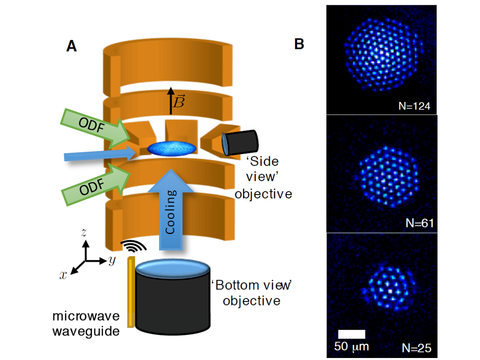An official website of the United States governmentOfficial websites use .gov
A .gov website belongs to an official government organization in the United States.Secure .gov websites use HTTPS
A lock ( ) or https:// means you’ve safely connected to the .gov website. Share sensitive information only on official, secure websites.

# Quantum Simulation and Sensing with Trapped Ions

## Summary

We use magnetic and electric fields in a Penning trap to confine two-dimensional Coulomb crystals of ions. We can perform quantum simulations of many-body spin Hamiltonians in these systems, and can perform sensing of electric fields below the standard quantum limit using quantum mechanical squeezing.

## Description(A) A cross-sectional illustration of the Penning trap (not to scale). The orange electrodes provide axial confinement and the rotating wall potential that controls the rotation of the array. The 4.5 T magnetic field is directed along the z axis. The blue disk indicates the 2D ion crystal. Resonant Doppler cooling is performed with the beams along z and y. The spin state–dependent optical dipole force (ODF) beams enter ±10° from the 2D ion plane.  Resonant microwave radiation for coupling ground states ↑ and ↓ is delivered through a waveguide.  (B) Coulomb crystal images in a frame rotating with the 9Be+ ions in ↑, with the number of ions N indicated.

Entanglement between individual quantum objects exponentially increases the complexity of quantum many-body systems, so systems with more than 30-40 quantum bits cannot be fully studied using conventional techniques and computers. To make progress at this frontier of physics, we are pursuing Feynman’s pioneering idea of quantum simulation with two-dimensional, single-plane arrays of trapped ions. We use a Penning ion trap (Fig. 1(A)), which employs static electric and magnetic fields for ion confinement, to form single-plane, 2-dimensional triangular arrays of several hundred 9Be+ ions (Fig. 1(B)). Trapped ions are naturally suited for simulating quantum magnetism. Long-range Ising interactions are engineered with spin-dependent forces on arrays up to ~300 ions. The spin-dependent forces couple the ion spins to a motional mode (or modes) of the ion crystal. At a basic level, the system we have engineered is the Dicke model,

$$\hat{H}^\mathrm{Dicke} = -\frac{g_0}{\sqrt{N}}(\hat{a}+\hat{a}^\dagger)\hat{S}_z + B(t)\hat{S}_x - \delta\hat{a}^\dagger\hat{a}$$

Where $$g_0$$ represents the homogeneous coupling between each ion and the axial center-of-mass mode, $$\hat{S}_\alpha, \alpha\in\{x, y, z\}$$ represents the composite spin of the $$N$$-ion crystal, $$B(t)$$ is the strength of a time-varying transverse field obtained through the application of microwaves resonant with the qubit frequency, and $$\delta$$ is the detuning of the frequency of the spin-dependent force from the frequency of the center-of-mass mode.

In this system we have benchmarked quantum dynamics and entanglement in single-plane crystals of greater than 200 ions through measurements of the composite magnetization (or spin) of the system  and through the measurement of out-of-time-order correlation functions that quantify the spread of quantum information throughout the system . Future goals include increasing the complexity of the system through the introduction of site-resolved addressing and exploring simulations and the generation of entangled spin states with three dimensional crystals where the number of ions can be greater than 10,000.

In related work we have studied the sensitivity with which weak motional excitations of the ion crystal center-of-mass mode can be measured.  This enables searches for weak electric fields, potentially from ultralight dark matter candidates.  The electric field sensitivity improves as $$N^{1/2}$$ where $$N$$ is the number of ions in the crystal.  Recent work demonstrated the ability to measure excitations (or displacements) of the 1.6 MHz center-of-mass motion of single-plane crystals with N~150 ions at a level of ~9 dB below the size of the ground-state wave function size in a single measurement . The enhanced sensitivity is obtained by preparing an entangled state of the ion spin and the center-of-mass motional degrees of freedom. Reading out the displaced entangled state is accomplished by reversing the interaction that produced the initial entangled state, resulting in an unentangled state where the ion spins are rotated by an amount proportional to the displacement. The angle of the ion spin-rotation can then be measured.

 J. G. Bohnet et al., “Quantum spin dynamics and entanglement generation with hundreds of trapped ions”, Science 352, 1297 (2016)

 M. Gärttner et al., "Measuring out-of-time-order correlations and multiple quantum spectra in a trapped ion quantum magnet", Nature Physics 13, 781 (2017)

 K. A. Gilmore, et al., “Quantum-enhanced sensing of displacements and electric fields with large trapped-ion crystals,” Science 373, 673 (2021).

## Personnel

Permanent staff: John Bollinger

Postdocs: Allison Carter, Jennifer Lilieholm